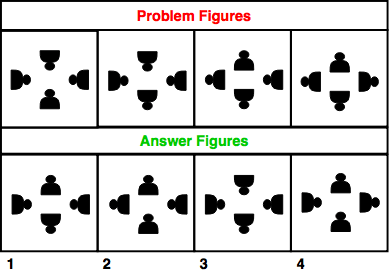# Non-Verbal Analogies - Logical Reasoning (MCQ) Questions for Q. 30062

Q.  The following question consists of some Problem Figures followed by other figures marked 1, 2, 3, 4 called the Answer Figures.

Find out the correct answer figure that should come next in the sequence of problem figures.- Published on 15 May 17

a. 1
b. 2
c. 3
d. 4

The element at the bottom is rotated through 180 degrees.

Then, two elements in the clockwise direction are rotated through 180 degrees.

In the third step, the element to the right is rotated through 180 degrees.

Thus, the next should be rotation of next two elements in the clockwise direction should be rotated by 180 degrees.

The answer figure should be figure 4.

## ➨ Post your comment / Share knowledgeEnter the code shown above:

(Note: If you cannot read the numbers in the above image, reload the page to generate a new one.)